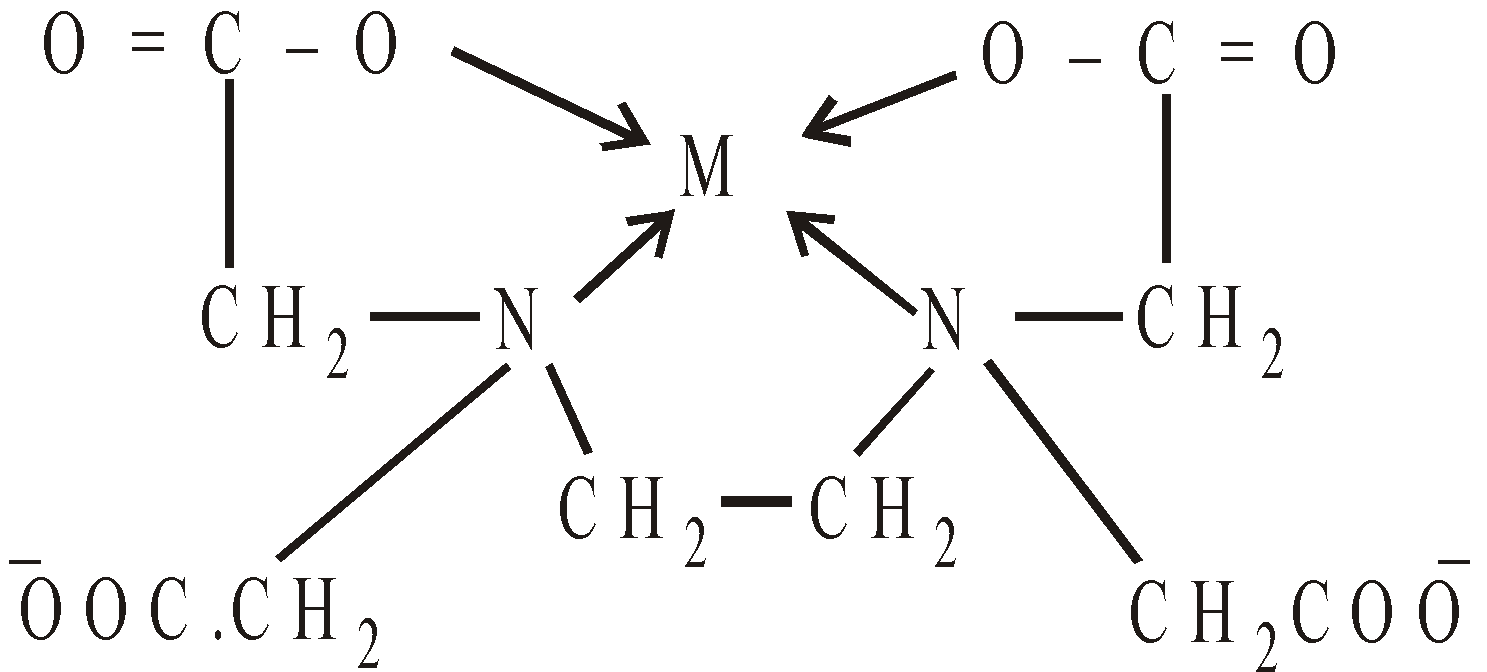# VOLUMETRIC ANALYSIS

### VOLUMETRIC METHODS

Volumetric or titrimetric analysis are quantitative analytical techniques which employ a titration in comparing an unknown with a standard. In a titration, a volume of a standardized solution containing a known concentration of reactant ‘A’ is added incrementally to a sample containing an unknown concentration of reactant  ‘B’ till reactant ‘B’ is just consumed (stoichiometric completion). This is known as the equivalence point.
At this point we have N1V1 = N2V2.

### INDICATOR

A compound added to the reacting solutions that undergo an abrupt change in a physical property usually a colour .

#### TYPES OF INDICATORS

• Internal - They are added to the reacting solutions
• External - Electrochemical devices such as pH meters

### END POINT

The point at which a titration is stopped i.e. equivalence point.

### STANDARD SOLUTION

The solution of known concentration of an acid, base or salt.

### EXAMPLES OF VOLUMETRIC METHODS

#### ACIDIMETRY AND ALKALIMETRY

Sodium Carbonate - Hydrochloric acid Titration
Reaction :Indicator : Methyl orange
End Point : Appearance of light pink colour

In presence of phenolphthalein the reaction between Na2CO3 and HCl is half complete#### PRECIPITATION TITRATIONS

• AgNO3 – NH4CNS OR KCNS TITRATION (VOLHARD'S METHOD)
Reaction :Slight excess of KCNS or NH4CNS gives blood red tinge with ferric salt.Indicator : Ferric alum solution
End Point : Appearance of blood red tinge
• AgNO3 - NaCl TITRATION (MOHR'S METHOD)
Reaction :Slight excess of AgNO3 gives a brick red ppt. with K2CrO4Indicator : Potassium Chromate solution
End Point : Appearance of brick red precipitate

#### REDOX TITRATIONS

OXALIC ACID - POTASSIUM PERMANGANATE TITRATION
Reaction :Indicator : KMnO4 is self indicator
End Point : Appearance of light pink colour

FERROUS AMM. SULPHATE - KMnO4 TITRATION
Reaction :Indicator : KMnO4 is self indicator
End Point : Appearance of light pink colour

FERROUS AMM. SULPHATE - K2Cr2O7 TITRATION
Reaction :Potassium ferricyanide gives blue coloured ppt. with ferrous ionsIndicator : Potassium ferricyanide (External) or Diphenylamine (internal).
End point : No green colour when K3[Fe(CN)6] is used.
Bluish violet or purple colour.
Whenis used.

I2-Na2S2O3.5H2O TITRATION
This is Iodometry titration. Since standard solution of iodine is used.
Reaction :Indicator : Freshly prepared starch solution.
End Point : Disappearance of blue colour.
Iodine is dissolved in KI solution and forms soluble KI3
KI + I2 KI3
Equilibrium shifts to left hand side when iodine is required.

As2O3-I2 TITRATION (IODOMETRY TITRATION)
Reaction :Solution of arsenious oxide is prepared in NaOH.;HI is removed by adding NaHCO3 to make reaction irreversible.Indicator : Freshly prepared starch solution.
End point : Disappearance of blue colour.

IODOMETRY
Iodine is liberated during chemical reactions.titration
Reaction :Indicator : Freshly  prepared starch solution
End point : Disappearance of blue colour.

K2Cr2O7-Na2S2O3.5H2O TITRATION (IODOMETRY)
Reaction :Indicator : Freshly prepared starch solution.
End point : Disappearance of blue colour.

#### COMPLEXATION REACTIONS

HARDWATER-EDTA TITRATION
Reaction :stable complexIndicator : Eriochrome black-T (Alcoholic solution)
End point :of water + Eriochrome  black-TThus wine red colour changes to blue at end point
• Acid base titration involves proton (H+) transfer reaction between analyte and titrant.
• Precipitation reactions, ions in solution combine to form an insoluble precipitate.
• Complexation reactions, analyte and titrant combine to form a very stable complex ion (held together by coordinate bonds)
• Redox reaction, electrons transfer between analyte and titrant.

### PRIMARY STANDARD

It is a reagent that is pure enough to be weighed out and used directly to provide a known number of moles eg. Na2CO3, FeSO4 (NH4)2SO4.6H2O, etc.

### STANDARD SOLUTION

A solution whose concentration is known. The concentration is generally expressed in terms of normality.

### EQUIVALENT MASS

It is the mass in gram which is chemically equivalent to 1.008 g of hydrogen, 8g of oxygen or 35.46 g of chlorine.
Equivalent mass of an acid=Equivalent mass of a base  =Equivalent weights of oxidising and reducing agents. On the basis of the change in O.N. of an element in the oxidising or reducing agent.
Eq. wt. =Thus in acidified KMnO4, Mn in ion is reduced to Mn2+, the change in O.N. is 5.
Hence equivalent weight of KMnO4 in acidified solution
Eq. wt. =In alkaline medium Mn in ion is reduced to MnO2, the change in O.N. is 3.
Hence equivalent weight of KMnO4 in alkaline medium.
Eq. wt. =Fe in FeSO4 is oxidised to Fe2(SO4)3, a change in O.N. is 1. Hence equivalent weight of FeSO4 is
Eq. Wt.  =In acidified solution Cr in K2Cr2O7, is reduced to Cr3+, the total change in O.N. is 6.
Hence equivalent weight of K2Cr2O7 is
Eq. wt. =Equivalent mass of a salt =### STRENGTH OF THE SOLUTION

It is expressed in grams per litre and related to normality and equivalent weight as,
Strength = Normality × equivalent weight.

#### RELATION BETWEEN NORMALITY AND MOLARITY

Normality = n × Molarity

where n = number of equivalents in 1 mole## Want to know more

Please fill in the details below:

## Latest NEET Articles\$type=three\$c=3\$author=hide\$comment=hide\$rm=hide\$date=hide\$snippet=hide

Name

ltr
item
BEST NEET COACHING CENTER | BEST IIT JEE COACHING INSTITUTE | BEST NEET & IIT JEE COACHING: Volumetric Analysis | Chemistry Notes for IITJEE/NEET
Volumetric Analysis | Chemistry Notes for IITJEE/NEET